# SSC CGL Tier 1 Exam Question Paper For 10 September 2016 Quantitative Aptitude

## SSC CGL Tier 1 Exam Question Paper For 10 September 2016 Quantitative Aptitude

SSC CGL Tier 1 Exam Question Paper For 10 September 2016 Quantitative Aptitude

EXAM DATE : 10 September 2016

EXAM NAME : SSC Examination 2016

TOTAL MARKS : 200

Question 51.A is twice as good as B and together they finish a piece of work in 16 days. The number of days taken by A alone to finish the work is

Options:

1) 20 days
2) 21 days
3) 22 days
4) 24 days

Question 52.The successive discount of 15%, 20% and 25% on an article is equivalent to the single discount of

Options:

1) 60%
2) 47%
3) 49%
4) 40%

Question 53.The numbers x, y, z are respectively proportional to 2, 3, 5 and the sum of x, y and z is 80. If the number z is given by the equation z = ax8,
then a is

Options:

1) 6
2) 3/2
3) 3
4) 5/2

Question 54.If the ratio of cost price and selling price be 10:11, then the profit percentage is

Options:

1) 1%
2) 10%
3) 5%
4) 8%

Question 55.The price of rice has increased by 60%. In order to restore the original price, the new price must be reduced by

Options:Question 56.A man covers a total distance of 100 km on bicycle. For the first 2 hours, the speed was 20 km/hr and for the rest of the journey, it came down to 10
km/hr. The average speed will be

Options:

1) km/hr
2) 13 km/hr
3) 15 1/8 km/hr
4) 20 Km/hr

Question 57.Alipta got some amount of money from her father. In how many years will the ratio of the money and the interest obtained from it be 10:3 at 6% simple
interest per annum?

Options:

1) 7 years
2) 3 years
3) 5 years
4) 4 years

Question 58.If (x 2) (x p) = x2 ax+ 6, then the value of (a p) is

Options:

1) 0
2) 1
3) 2
4) 3

Question 59.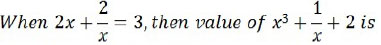Options:

1) 2/7
2) 7/8
3) 7/2
4) 8/7

Question 60.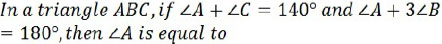Options:

1) 80°
2) 40°
3) 60°
4) 20°

Question 61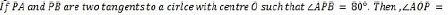Options:

1) 40°
2) 50°
3) 60°
4) 70°

Question 62.If tan (5x 10°) = cot (5y + 20°), then the value of x + y is

Options:

1) 15°
2) 16°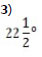4) 24°

Question 63.Which one of the following is the minimum value of the sum of two integers whose product is 24?

Options:

1) 25
2) 11
3) 8
4) 10

Question 64.The average marks obtained by a class of 60 students is 65. The average marks of half of the students is found to be 85. The average marks of the
remaining students is

Options:

1) 35
2) 45
3) 55
4) 65

Question 65.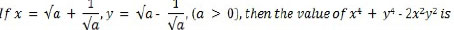Options:

1) 16
2) 20
3) 10
4) 5

Question 66.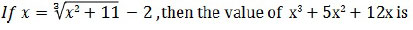Options:

1) 0
2) 3
3) 7
4) 11

Question 67.Which of the set of three sides can’t form a triangle?

Options:

1) 5 cm, 6 cm, 7 cm
2) 5 cm, 8 cm, 15 cm
3) 8 cm, 15 cm, 18 cm
4) 6 cm, 7 cm, 11 cm

Correct Answer: 5 cm, 8 cm, 15 cm

Question 68.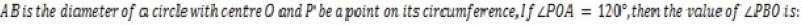Options:

1) 30°
2) 60°
3) 50°
4) 40°

Question 69.A pilot in an aeroplane at an altitude of 200 m observes two points lying on either side of a river. If the angles of depression of the two points be 45° and
60°, then the width of the river is

Options: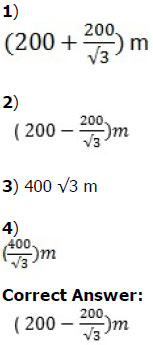Question 70.A solid sphere of radius 9 cm is melted to form a sphere of radius 6 cm and a right circular cylinder of same radius. The height of the cylinder so formed
is

Options:

1) 19 cm
2) 21 cm
3) 23 cm
4) 25 cm

Question 71.An arc of 30° in one circle is double an arc in a second circle, the radius of which is three times the radius of the first. Then the angles subtended by the
arc of the second circle at its centre is

Options:

1) 3°
2) 4°
3) 5°
4) 6°

Study the Bar diagram carefully and answer the questions. The Bar diagram shows the trends of foreign direct investment (FDI) into India from all over the World (in Rs. crores).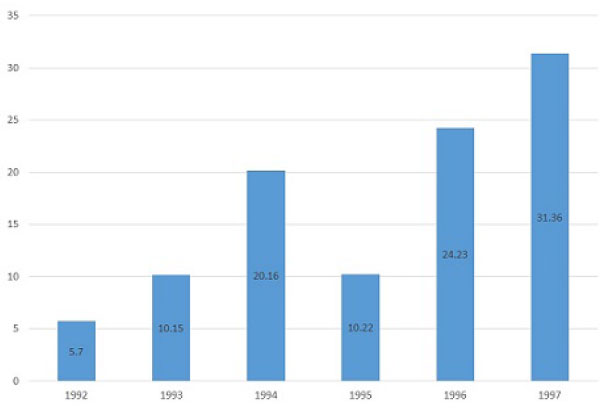Question 72. The sum of FDI of 1992 and 1993 is

Options:

1) 15.58 cr
2) 15.85 cr
3) 15.22 cr
4) 15.65 cr

Question 73. The year which exhibited the 2nd highest growth percentage in FDI in India over the period shown is

Options:

1) 1993
2) 1994
3) 1997
4) 1996

Question 74. The ratio of investment in 1997 to the average investment is

Options:

1) 2:1
2) 1:2
3) 1:1
4) 3:1